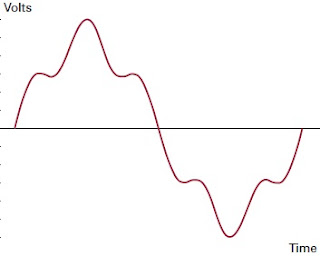# What are harmonics?

A harmonic is define as a component of a periodic wave having a frequency that is an integral multiple of the fundamental power line frequency of 60 Hz.Fig: 1 Fundamental and 5th HarmonicFig: 2 voltage wave distortion

For example, 300 Hz (5 x 60 Hz) is a 5th order harmonic of the fundamental frequency (Figure 1) . Figure 2 shows the resultant wave when the fundamental and 5th harmonic are combined .

The result is harmonic distortion of the power waveform . Harmonics typically seen on a power system can be subdivided into two distinct categories by the nature of the problems they create and the remedies they usually require .

Those harmonic currents that are the dominant harmonic orders created by three-phase nonlinear loads—5th, 7th, 11th, 13th, and higher order odd harmonics that are not multiples of three

Those harmonics created primarily by single-phase nonlinear loads—3rd order harmonics and higher multiples of three.

These are sometimes referred to as triplet or zero-sequence harmonics and are usually accompanied by some 5th, 7th, and other higher order harmonics

Harmonics are a steady-state phenomenon and should not be confused with short-term phenomena that last less than a few cycles.

Transients, electrical disturbances, overvoltage surges, and undervoltage sags in the supplied voltage are not harmonics.

Some of these short-term disturbances in voltage or current can be mitigated by transient voltage surge suppressors, line reactors, or isolation transformers. However, these devices usually have little, if any, effect on harmonic currents or voltages.

The level of voltage or current harmonic distortion existing at any one point on a power system can be expressed in terms of the total harmonic distortion (THD) of the current or voltage waveform.

The THD (for a voltage waveform) is given by the following formula:THD (for a voltage waveform)

## How are harmonics generated?

Harmonics are generated by nonlinear loads.A nonlinear load is a circuit element that draws current in a nonsinusoidal manner. Until recently, most factory loads were primarily linear, with current waveform closely matching the sinusoidal voltage waveform and changing in proportion to the load.

More recently, however, factory loads with major nonlinear components have increased dramatically.

Nonlinear devices that cause the most problems can generally be grouped into two categories electronic power converters and arcing devices.

### Electronic power converters

Electronic power converters—for example, adjustable speed drives and power supplies—are by far the largest contributors to harmonic distortion in today’s plant environment .

An electronic power converter changes electrical energy from one form to another, typically by rectifying the AC voltage into DC and utilizing the DC voltage directly or synthesizing a new AC voltage.

This change is accomplished by using solid-state devices—silicon control rectifiers (SCRs), diodes, transistors—to periodically switch in the conducting circuits of the converter.

Below are some common names that are typically associated with electronic power converters .

#### Common names given to electronic power converters

Variable frequency drives
• SCR drives
AC motor drives (AC/DC/AC)
• DC motor drives (AC/DC)
• Three-phase full wave rectifiers
• Three-phase full wave converters
• Six-pulse converters
As most plant engineers appreciate, there is an increased use of electronic drives due to their ability to more efficiently or precisely drive a motor or process .

### Arcing devices

Arc furnaces and welders are the two types of arcing devices that cause the most harmonic distortion, although arc lighting (fluorescent, mercury vapor) will also cause small degrees of harmonic distortion .

### Other equipment

Motors, generators, transformers, and arc lighting also have small nonlinear components, although the contribution of these devices to total harmonic distortion in a plant tends to be relatively small .

# Effect of harmonics

### Resonance

The use of both capacitive and inductive devices in distribution systems leads to resonance phenomena, resulting in extremely high or low impedance values. These variations in impedance modify the current and voltage in the distribution system. Here we will discuss only parallel-resonance phenomena, which are the most frequent.
Consider the simplified diagram below, showing an installation made up of:

• a transformer supplying power,
• non-linear loads causing harmonic currents,
• power factor correction capacitors.

Resonance occurs when the denominator 1-LsCω2 approaches zero. The corresponding frequency is called the resonant frequency of the circuit.

At this frequency, the impedance is at its maximum value, resulting in considerable voltage harmonics and consequently major voltage distortion.

This voltage distortion is accompanied by the circulation of harmonic currents in the Ls + C circuit which are greater than the injected harmonic currents.

The distribution system and the power factor correction capacitors are subjected to considerable harmonic currents, resulting in the risk of overloads.

### Increased losses

#### 1) Losses in conductors

The active power transmitted to a load depends on the fundamental current. When the current drawn by the load contains harmonics, the rms value of the current (Iᵣₘₛ) is greater than the fundamental I₁
Current harmonics provoke an increase in Joule losses in all the conductors through which they flow and additional temperature rise in the transformers, circuit breakers, cables, etc.THD

#### 2) Losses in asynchronous machines

Voltage harmonics, when applied to asynchronous machines, provoke the flow of currents with frequencies higher than 50 Hz in the rotor. These currents cause additional losses that are proportional to Uh² /h.

#### 3) Losses in capacitors

Harmonic voltage, when applied to capacitors, provokes the flow of currents that are proportional to the frequency of the harmonics. These currents cause additional losses.